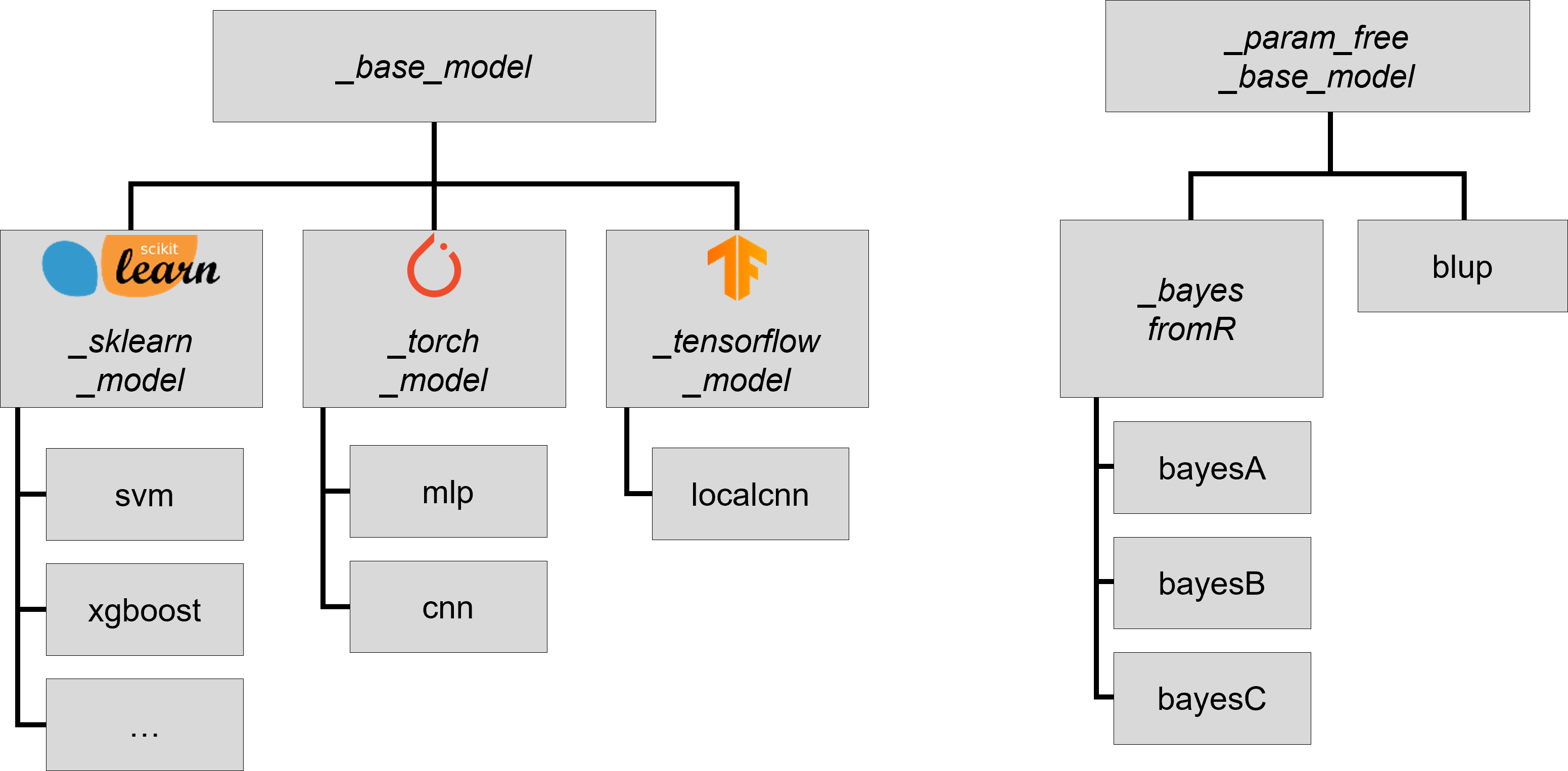# HowTo: Integrate your own prediction model

In this tutorial, we will show you how to integrate your own new prediction model into easyPheno using an example. We recommend to first watch the Code walkthrough video for a better understanding of easyPheno’s structure.

We further recorded a Video tutorial: Integrate new model, which is embedded below .

## Overview

The design of the model class makes easyPheno easily extendable with new prediction models. The subsequent figure gives an overview on its structure.All prediction models are either based on BaseModel or ParamFreeBaseModel in case your model does not contain hyperparameters for optimization (or you do not want to optimize any). These two base models define some methods that are common for all prediction models as well as all methods that each prediction model needs to implement. In this tutorial, we focus on BaseModel. easyPheno already contains child classes of BaseModel implementing some of its obligatory methods for TensorFlow, PyTorch and sklearn. As a consequence, adding a new prediction model based on one of these three very common machine learning frameworks only requires the definition of two attributes and implementation of two methods, which makes easyPheno easy extendable.

## An example: Integrating k-nearest-neighbors

We provide template files for all three frameworks and focus on TemplateSklearnModel for the remainder of this tutorial:

```import sklearn

from . import _sklearn_model

class TemplateSklearnModel(_sklearn_model.SklearnModel):
"""
Template file for a prediction model based on :obj:`~easypheno.model._sklearn_model.SklearnModel`

1. Copy this template file and rename it according to your model (will be the name to call it later on on the command line)

2. Rename the class and add it to *easypheno.model.__init__.py*

3. Adjust the class attributes if necessary

4. Define your model in *define_model()*

5. Define the hyperparameters and ranges you want to use for optimization in *define_hyperparams_to_tune()*

6. Test your new prediction model using toy data
"""
standard_encoding = ...
possible_encodings = [...]

def define_model(self):
"""
Definition of the actual prediction model.

Use *param = self.suggest_hyperparam_to_optuna(PARAM_NAME_IN_DEFINE_HYPERPARAMS_TO_TUNE)* if you want to use
the value of a hyperparameter that should be optimized.
The function needs to return the model object.

"""
...

def define_hyperparams_to_tune(self) -> dict:
"""
Define the hyperparameters and ranges you want to optimize.
Caution: they will only be optimized if you add them via *self.suggest_hyperparam_to_optuna(PARAM_NAME)* in *define_model()*

"""
return {
'example_param_1': {
'datatype': 'categorical',
'list_of_values': ['cat', 'dog', 'elephant']
},
'example_param_2': {
'datatype': 'float',
'lower_bound': 0.05,
'upper_bound': 0.95,
'step': 0.05
},
'example_param_3': {
'datatype': 'int',
'lower_bound': 1,
'upper_bound': 100
}
}
```

As an example, we will integrate k-nearest-neighbors (knn) as a new prediction model, both for classification and regression.

First, we copy the template file into the folder containing easyPheno’s subpackage model and rename it to knn.py. Further, we rename the class within the file to `Knn` and add `"knn"` to `__all__` in easypheno.model.__init__.py.

So with updated comments (including `:obj:` references for linking in the auto-generated API documentation), our file now contains the following code:

```import sklearn

from . import _sklearn_model

class Knn(_sklearn_model.SklearnModel):
"""
Implementation of a class for k nearest neighbours regressor respective classifier.

"""
standard_encoding = ...
possible_encodings = [...]

def define_model(self):
"""
Definition of the actual prediction model.

"""
...

def define_hyperparams_to_tune(self) -> dict:
"""
Definition of hyperparameters and ranges to optimize.

"""
...
```

Now we need to define the two attributes and implement the two methods. We will use the standard `'012'` encoding in this case (see here for information on the encodings). Further, we optimize the two hyperparameters `n_neighbors` and `weights`. These need to be suggested to Optuna via `self.suggest_hyperparam_to_optuna(PARAM_NAME` in `define_model()` and defined with their ranges in `define_hyperparams_to_tune()` (see here for more information regarding the format and possible options for hyperparameter definition). Finally, we distinguish between `'classification'` and `'regression'` by using the inherited attribute `self.task`.

```import sklearn

from . import _sklearn_model

class Knn(_sklearn_model.SklearnModel):
"""
Implementation of a class for k nearest neighbours regressor respective classifier.

"""
standard_encoding = '012'
possible_encodings = ['012']

def define_model(self):
"""
Definition of the actual prediction model.

"""
n_neighbors = self.suggest_hyperparam_to_optuna('n_neighbors')
weights = self.suggest_hyperparam_to_optuna('weights')
return sklearn.neighbors.KNeighborsClassifier(n_neighbors=n_neighbors, weights=weights)
else:
return sklearn.neighbors.KNeighborsRegressor(n_neighbors=n_neighbors, weights=weights)

def define_hyperparams_to_tune(self) -> dict:
"""
Definition of hyperparameters and ranges to optimize.

"""
return {
'n_neighbors': {
'datatype': 'int',
'lower_bound': 2,
'upper_bound': 50,
'step': 2
},
'weights': {
'datatype': 'categorical',
'list_of_values': ['uniform', 'distance']
}
}
```

Now we are able to test our new prediction model with toy data by calling `python3 -m easypheno.run` with the option `-mod knn` (see HowTo: Run easyPheno using Docker).

This example gives an overview on how to integrate your own prediction model. Feel free to get guidance from existing prediction models as well. We are always happy to welcome new contributors and appreciate if you help improving easyPheno by providing your prediction model.